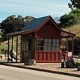# Gas station without pumps

## 2014 February 1

### More on segmenting noisy signals

Filed under: Uncategorized — gasstationwithoutpumps @ 10:34
Tags: , ,

Last August, I posted on Segmenting noisy signals from nanopores, but I’ve always been a bit unsatisfied with the parameterization of that method.  The basic idea is to have a parameterized probabilistic model for a segment, Pθ(s:t), where θ is some parameter setting for the model optimized for the half-open time interval [s,t).  We compare the probability of the segment modeled as two subsegments with the segment modeled as a single unit:$L_{i}=\log \frac{P_{\theta_{1}}(s:i) P_{\theta_{2}}(i:t)}{P_{\theta_{0}}(s:e)} = \log P_{\theta_{1}}(s:i) + \log P_{\theta_{2}}(i:t) - \log P_{\theta_{0}}(s:t)$,

where i is the breakpoint, θ1 and θ2 are the parameters for the two segments, and θ0 is the parameter setting for the whole segment.  I showed in the previous post how to simplify this for stepwise Gaussian models to$L_{i} = (s-i)\ln \sigma_{1} + (i-t)\ln \sigma_{2} - (s-t)\ln \sigma_{0}$.  Note that translation or scaling of the samples will not affect this log-likelihood, as the means have already cancelled, and scaling adds a constant to all the$\ln\sigma$ values, and that constant also cancels.

To find the best breakpoint in an interval, we check each possible breakpoint, and pick the one that maximizes Li. We then decide whether that Li is big enough to keep the breakpoint or not using a user-settable threshold. If the threshold is set very low, then the user is saying that there is not much noise and we should follow the signal closely. If the threshold is set high, then the user is saying that there is a lot of noise and we need to be very confident of any breakpoint we claim.

What has been bothering me is how we’ve been setting the threshold.  I’d really like to have an easily interpreted parameter that we can set independent of sampling rate or other confounding factors.

I started out by looking at how$L_{i}$ is distributed for sequences that are generated from a single Gaussian model (with no step).  I did a bunch of simulation experiments, and I was surprised to find that the probability density function appears to be a simple exponential distribution$Pr(L_{i} \geq x ) = e^{-x}$ for$x\geq 0$, and that this distribution does not depend on how big a sequence is being split.

That lack of dependence on how big the sequence is means that I don’t have to adjust the threshold based on how big a window I’m looking at. I had originally thought that there ought to be such an adjustment, but I got better results by keeping the threshold fixed.

If the empirically observed exponential distribution of$L_{i}$ is correct, then we can parameterize the threshold in terms of a false-positives-per-second parameter, F. If we divide by the sampling frequency, we get a false-positive-per-sample$F/f_{s}$. The threshold T needs to be set so that$Pr(L_{i} \geq T ) = e^{-T} \leq F/f_{s}$, or$T\geq -\ln(F/f_{s})$.

But specifying a tolerance for false positives is not good enough, as one generally wants about the same false positive rate independent of how fast the signal is changing, but the threshold should be tighter if you expect the signal to remain constant for a long time, and looser if you want to track the signal changes rapidly.  I think that this can be handled by not using the likelihood ratio, but the posterior odds ratio, in a properly Bayesian way.  The user of the segmenter expresses how finely they want things divided in terms of expected steps per second, sps, as well as an acceptable overcalling rate, over, (say 1% of segments getting split), which sets the false-positive rate F=sps*over.

The prior probability of a step at each sample is$\mbox{\it sps}/f_{s}$, so the prior odds ratio is$\frac{ \mbox{\it sps} }{f_{s}-\mbox{\it sps}}$, and the posterior odds ratio is$L_{i} \frac{ \mbox{\it sps} }{f_{s}-\mbox{\it sps}}$.  The threshold on likelihood would then be set to$T=-\ln \frac{ \mbox{\it sps} }{f_{s}-\mbox{\it sps}}-\ln(F/f_{s})\approx -2\ln(\mbox{\it sps}) -\ln(\mbox{\it over})+2\ln(f_{s})$.

On the sample event that I’ve been using for testing, setting the segments/second to 10, and the oversegmentation rate to 0.01 gets me pretty much the segmentation I want to see, with an average segment length of 68.79ms (14.5 segments per second).  Adjusting the prior segments/second to 1.0 gets me 11.6 segments per second, and to 100.0 gets me 18.4 segments/second, so the prior is having about the right effect on the segmentation, adjusting the rate of segmentation without overwhelming the data.  Since the underlying segmenter is the same as before (only the parameters used for setting the threshold have changed),  it may still be difficult to find settings that work for some nanopore experiments.

1.[…] noisy signals from nanopores, and in January I updated that with improved parameterization in More on segmenting noisy signals.  Last week, Jacob Schreiber (the programmer for the nanopore group) found some problems with the […]

Pingback by Segmenting filtered signals | Gas station without pumps — 2014 May 24 @ 14:27

2.[…] More on segmenting noisy signals. […]

Pingback by Segmenting noisy signals revisited | Gas station without pumps — 2014 June 17 @ 20:16

This site uses Akismet to reduce spam. Learn how your comment data is processed.